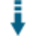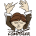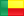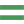# PHP Complex Numbers class: Perform arithmetic operations with complex numbersDownload .zip
 InfoExampleView files (4)Download .zip Reputation Support forum Blog Links
 Ratings Unique User Downloads Download Rankings Not enough user ratings Total: 139 All time: 9,191 This week: 189Version License PHP version Categories m2 1.0.0 Custom (specified... 5 PHP 5, Math
Description

Author

This package can perform arithmetic operations with complex numbers.

It can store a complex number value with real and imaginary parts.

The class can perform several types of complex number operation like:

- SQuare Root (sqrt)
- Exponentiation (pow)
- Inversion
- Absolute value (abs)
- Addition, subtraction, multiplication and divisionPerformance Level
Name: Classes: zinsou A.A.E.Mo�se is available for providing paid consulting. Contact zinsou A.A.E.Mo�se . 50 packages by zinsou A.A.E.Mo�seBenin 34 681 1 in Benin1421 in BeninInnovation awardNominee: 23x

Winner: 2x

## Example

 ``` '; echo '

exponential form:

'; var_dump(\$a=new Arcomplex('5.6111611111-3.59I')); var_dump(\$exp=\$a->Cexponentialform()); var_dump(exptocomplex(\$exp)); var_dump(exptocomplex("6.6613233681245*(e(-0.56916865378565*i))")); var_dump(exptocomplex("6.6613233681245(e(-0.56916865378565i))")); var_dump(exptocomplex("6.6613233681245.(e(-0.56916865378565.i))")); echo '

Complex power:

'; \$c=new Arcomplex('0.001+0.6533I'); var_dump(\$c->Cpow(-2)); var_dump(\$c->Cpow(2)); \$x=new COP(new Arcomplex(\$c->Cpow(-10)),new Arcomplex(\$c->Cpow(10))); var_dump(\$x->Cmul()); \$x=new COP(new Arcomplex('1+3I'),new Arcomplex('1-3I')); echo '

Complex division and multiplication:

'; var_dump(\$x->Cdiv()); var_dump(\$x->Cmul()); \$x=new COP(new Arcomplex('1-1.5i'),new Arcomplex('1-i')); var_dump(\$x->Cdiv()); var_dump(\$x->Cmul()); echo '

Complex inverse and opposite form:

'; var_dump(\$a=new Arcomplex('-2+2I')); var_dump(\$e=\$a->Cinv()); var_dump(\$e->Cform()); var_dump(\$e=\$a->Copp()); var_dump(\$e->Cform()); echo '

Complex square root:

'; var_dump(\$a=new Arcomplex('-4')); var_dump(\$e=\$a->Csqrt()); var_dump(\$e->Cform()); \$x=new COP(new Arcomplex(\$e->Cform()),new Arcomplex(\$e->Cform())); var_dump(\$x->Cmul()); var_dump(\$a=new Arcomplex(array(-4,6))); var_dump(\$e=\$a->Csqrt()); var_dump(\$e->Cform()); \$x=new COP(new Arcomplex(\$e->Cform()),new Arcomplex(\$e->Cform())); var_dump(\$x->Cmul()); var_dump(\$a=new Arcomplex(array('Im'=>-4,'Re'=>6))); var_dump(\$e=\$a->Csqrt()); var_dump(\$e->Cform()); \$x=new COP(new Arcomplex(\$e->Cform()),new Arcomplex(\$e->Cform())); var_dump(\$x->Cmul()); echo '

Complex all square roots:

'; var_dump(\$a=new Arcomplex(array(0,-0.001))); var_dump(\$e=\$a->AllCsqrt()); var_dump(\$e->Cform()); var_dump(\$e->Cform()); \$x=new COP(new Arcomplex(\$e->Cform()),new Arcomplex(\$e->Cform())); var_dump(\$x->Cmul()); \$x=new COP(new Arcomplex(\$e->Cform()),new Arcomplex(\$e->Cform())); var_dump(\$x->Cmul()); echo '
';  ```

## Details

 ```ArComplex.class.package is a package for handling complex arithmetic on/with PHP Language. This class allows users to do all human can do on or with complex numbers. This class is very easy to use and also very easy to understand. ArComplex.class.package allows users to specify his complex number just like an array example: \$a=new Arcomplex(array(-4,6)); where the first element is real and the second imaginary... or example:\$a=new Arcomplex(array('Im||im||i||I||imaginary'=>-4,'Re||re||r||R||real'=>6))); here the order doesn't count or as a numeric for complex number with only real part just as example \$a=new Arcomplex(-4); or even more easily as a string: example: \$c=new Arcomplex('0.001+0.6533I'); or \$c=new Arcomplex('0.001+0.6533i'); or \$c=new Arcomplex('0.001+I'); or \$c=new Arcomplex('0.001i');//the last example is a complex with just an imaginary part..... and so one.... For other examples, see the 'complextest.php' file or read the class declaration to understand... The package contains two classes: one for basics operations on one complex number and one other for operations between two complex number... For more informations or bug reporting or suggestions Please contact me at this e-mail leizmo@gmail.com ```Files
File Role DescriptionArComplex.class.php Class ArComplex.classcomplextest.php Example testlicense Lic. licensereadme Doc. readme

 All time: 9,191 This week: 189About usAdvertise on this siteSite mapNewsletterStatisticsSite tipsPrivacy policyContact
For more information send a message to `info at phpclasses dot org`.